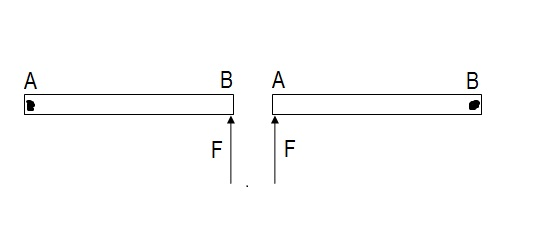# Silly rods

The figure below shows a non uniform metre stick AB having the linear mass density variation $\lambda=\lambda_{o}x$ where $\lambda_{o}$ is a constant and $x$ is the distance from end $A$, placed on smooth horizontal surface.

In the first experiment, the rod is pivoted at $A$ and force $F$ is applied perpendicular to the rod at the other end $B$ and in the second experiment, the rod is pivoted at $B$ and the force is applied perpendicular to the rod at the end $A$.

If in the first case angular acceleration is $\alpha_{A}$ and in the second case it is $\alpha_{B}$ thenRefer to my set

×